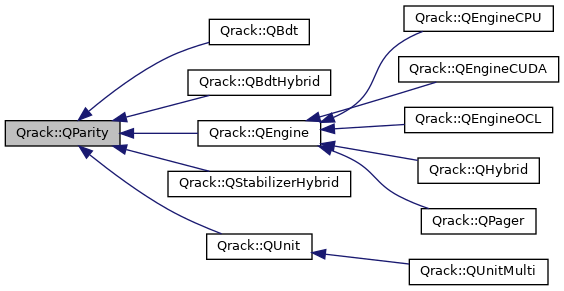Qrack  7.0 General classical-emulating-quantum development framework
Qrack::QParity Class Referenceabstract

#include <qparity.hpp>

Inheritance diagram for Qrack::QParity:[legend]

## Public Member Functions

Measure (and collapse) parity of the masked set of qubits. More...

virtual void UniformParityRZ (bitCapInt mask, real1_f angle)
If the target qubit set parity is odd, this applies a phase factor of $$e^{i angle}$$. More...

Overall probability of any odd permutation of the masked set of bits. More...

virtual bool ForceMParity (bitCapInt mask, bool result, bool doForce=true)=0
Act as if is a measurement of parity of the masked set of qubits was applied, except force the (usually random) result. More...

virtual void CUniformParityRZ (const bitLenInt *controls, bitLenInt controlLen, bitCapInt mask, real1_f angle)=0
If the controls are set and the target qubit set parity is odd, this applies a phase factor of $$e^{i angle}$$. More...

## ◆ CUniformParityRZ()

 virtual void Qrack::QParity::CUniformParityRZ ( const bitLenInt * controls, bitLenInt controlLen, bitCapInt mask, real1_f angle )
pure virtual

If the controls are set and the target qubit set parity is odd, this applies a phase factor of $$e^{i angle}$$.

If the controls are set and the target qubit set parity is even, this applies the conjugate, $$e^{-i angle}$$. Otherwise, do nothing if any control is not set.

## ◆ ForceMParity()

 virtual bool Qrack::QParity::ForceMParity ( bitCapInt mask, bool result, bool doForce = true )
pure virtual

Act as if is a measurement of parity of the masked set of qubits was applied, except force the (usually random) result.

Warning
PSEUDO-QUANTUM

## ◆ MParity()

 virtual bool Qrack::QParity::MParity ( bitCapInt mask )
inlinevirtual

Measure (and collapse) parity of the masked set of qubits.

## ◆ ProbParity()

 virtual real1_f Qrack::QParity::ProbParity ( bitCapInt mask )
pure virtual

Overall probability of any odd permutation of the masked set of bits.

## ◆ UniformParityRZ()

 virtual void Qrack::QParity::UniformParityRZ ( bitCapInt mask, real1_f angle )
inlinevirtual

If the target qubit set parity is odd, this applies a phase factor of $$e^{i angle}$$.

If the target qubit set parity is even, this applies the conjugate, e^{-i angle}.

Reimplemented in Qrack::QStabilizerHybrid, Qrack::QMaskFusion, Qrack::QEngineOCL, Qrack::QEngineCPU, Qrack::QHybrid, and Qrack::QPager.

The documentation for this class was generated from the following file: